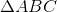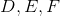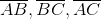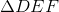# ISEE Upper Level Quantitative : How to find the area of an equilateral triangle

## Example Questions

### Example Question #1 : Equilateral Trianglesis an equilateral triangle. Pointsare the midpoints of, respectively.is constructed.

Which is the greater quantity?

(a) The area of(b) Twice the area ofIt is impossible to tell from the information given.

(a) and (b) are equal.

(a) is greater.

(b) is greater.

If segments are constructed in which the endpoints form the midpoints of the sides of a triangle, then four triangles, congruent to each other and similar to the larger triangle, are formed. Therefore, one of these triangles - specifically,- would have one-fourth the area of. This meanshas more than twice the area of.